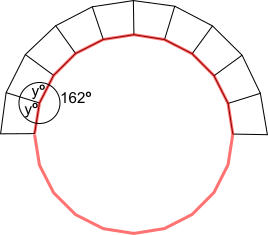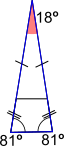You may also likeGetting an Angle

How can you make an angle of 60 degrees by folding a sheet of paper twice?Arclets Explained

This article gives an wonderful insight into students working on the Arclets problem that first appeared in the Sept 2002 edition of the NRICH website.Bow Tie

Show how this pentagonal tile can be used to tile the plane and describe the transformations which map this pentagon to its images in the tiling.

Trapezium Arch

Age 11 to 14 ShortChallenge Level

Sachdave from Doha College in Qatar used exterior angles, marked $\text a$ in the diagram below. Sachdave's method was similar to this:Tracing around the arch, it is necessary to turn through $\text{a}^\text{o}$ nine times, or ten times to end up facing in the opposite direction from where you started. You would also turn through $180^\text{o}.$

So $\text a = 180\div10 = 18$. Then notice that $\text{a, } x$ and ${x}$ make a straight line, so $\text a + 2x = 180,$ so $2 x = 162,$ so $x = 81.$

Sameer from Hymers College and Kira and Emma from Wycombe High School in the UK considered the angles in a regular icosagon (twenty-sided polygon). Sameer said:The inner perimeter of this shape forms half of a regular icosagon.

In any regular polygon, the sum of the exterior angles is $360^\text{o}.$ As there are $20$ exterior angles, each one is $360^\text{o}\div20$, which is $18^\text{o}.$

The interior angle is $180^\text o-$ exterior angle, which will be $180^\text o-18^\text o=162^\text o.$ This is shown in the diagram.

The interior angle forms a circle with the smaller angles of the trapeziums. In a circle, all the angles add up to $360^\text o.$

The two angles marked $y^\text o$ intersecting this circle are the same, so \begin{align}360&=162+2y\\ \Rightarrow 198&=2y\\ \Rightarrow y&=99\end{align}

In a trapezium, the angles add up to $360^\text o,$ so \begin{align}2x+2y&=360\\ \Rightarrow x+y&=180\\ \Rightarrow x+99&=180\\ \Rightarrow x&=81\end{align}

Sophie from the Stephen Perse Foundation used triangles:$$180^\text{o}\div10=18^\text o$$Each of the triangles is isosceles.
$\dfrac{180-18}{2}=81$ which is shown on the diagram.

Angles on a straight line add up to $180^\text o$
$180-81=99$ which is shown on the diagram.

Considering the trapezium below the triangle,
\begin{align}99\times2&=198\\\frac{360-198}{2}&=81\end{align}

Sophie could also have used the larger isosceles triangles directly:Thank you also to Toby from Chairo Christian School in Australia, who submitted a correct solution.

This problem is taken from the UKMT Mathematical Challenges.
You can find more short problems, arranged by curriculum topic, in our short problems collection.